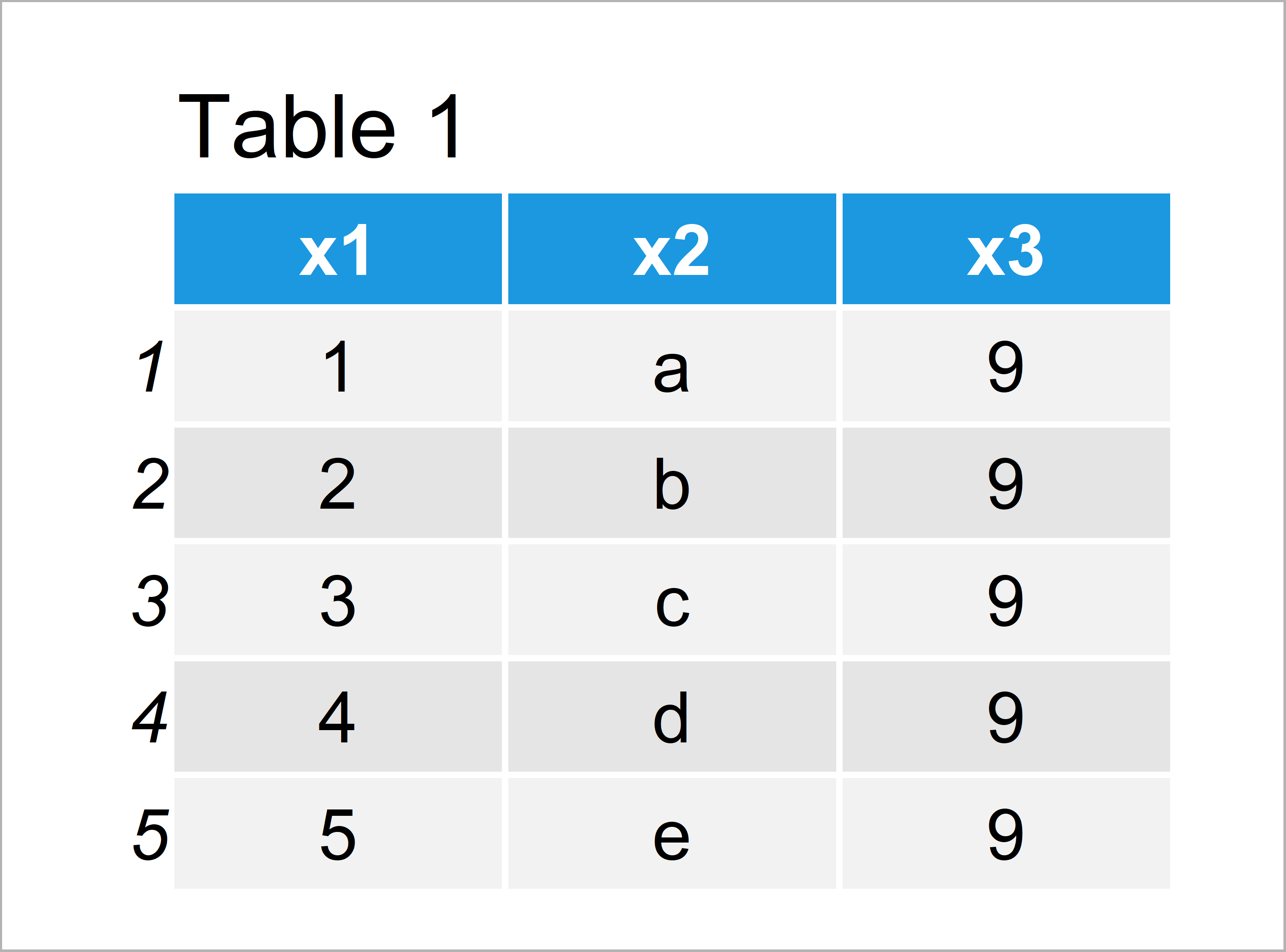# Meaning of \$ Operator in R (2 Examples)

In this tutorial you’ll learn how to use the \$ operator in the R programming language.

The content of the page is structured as follows:

Let’s start right away!

## Example 1: Using \$ Operator to Access Data Frame Column

Generally speaking, the \$ operator is used to extract or subset a specific part of a data object in R. For instance, this can be a data frame object or a list.

In this example, I’ll explain how to extract the values in a data frame columns using the \$ operator.

In preparation for the example, we have to create a data frame in R. We can do that by executing the following R syntax:

```data <- data.frame(x1 = 1:5,    # Create example data
x2 = letters[1:5],
x3 = 9)
data                            # Print example data```As shown in Table 1, we have created a data frame with five rows and three columns with the previous R syntax. The names of our variables are x1, x2, and x3.

Let’s assume that we want to extract the second column of our data frame, i.e. the variable x2. Then, we can use the \$ operator as shown below:

```data\$x2                         # Extract column of data
#  "a" "b" "c" "d" "e"```

Have a look at the previous output of the RStudio console. As you can see, we have extracted only the values stored in the variable x2.

## Example 2: Using \$ Operator to Access Element of List

As already mentioned, it is also possible to use the \$ operator to return specific list elements. To illustrate that, we first have to create an example list in R:

```my_list <- list(A = 1:5,        # Create example list
B = letters[1:5],
C = 9)
my_list                         # Print example list
# \$A
#  1 2 3 4 5
#
# \$B
#  "a" "b" "c" "d" "e"
#
# \$C
#  9
#```

The previous output of the RStudio console shows the structure of our list. Our list consists of three list elements that are called A, B, and C. Each of these list elements contains different values.

Let’s assume that we want to return only the values of the list element B. In this case, we can use the \$ operator as shown below:

```my_list\$B                       # Extract element of list
#  "a" "b" "c" "d" "e"```

The previous output of the RStudio console shows only the values that are contained in the second list element B.

## Video, Further Resources & Summary

In case you need further information on the R programming codes of this tutorial, you could watch the following video of the Statistics Globe YouTube channel. In the video, I’m explaining the contents of this article:

Please accept YouTube cookies to play this video. By accepting you will be accessing content from YouTube, a service provided by an external third party.If you accept this notice, your choice will be saved and the page will refresh.

Furthermore, you may want to have a look at the related articles which I have published on this website.

In summary: In this tutorial, I have illustrated how to apply the \$ operator in R. Don’t hesitate to let me know in the comments section below, if you have additional questions.

Subscribe to the Statistics Globe Newsletter

•December 22, 2021 12:33 pm

Hi Joachim,

Lovely seeing your blog! I have a question specifically for the second example. Do you think it’s possible to use the \$ operator to select multiple list elements? So for example, instead of my_list\$B only, you include A and B

Thanks,

•December 22, 2021 2:02 pm

Thanks a lot for the kind words, glad you like my website!

Regarding your question: I would use the following R code to extract multiple list elements:

`my_list[c("A", "B")]`

Regards,
Joachim

•skyrock
October 3, 2022 10:17 am

thank you bro, it was so helpfull

•October 5, 2022 8:51 am

Hey,

This is great to hear, glad it helped!

Regards,
Joachim

•soha
January 5, 2023 10:58 pm

can i use \$ to combine variables of unequal length to a data?

i have 3 variables of different lengths
and they are factors
length(x) = 2 (factor with 2 levels)
length(y)=2 (factor with 2 levels)
lenght(f)=5 ( factor of 5 levels and 4 values)
and the data which is file (p.txt) contain 4403
every time i try to add this variable as columns to the data it give me error of replacment due to the different length

how to combine them to the data file without losing their type as factors

i tried maxlength but it convert them to integer
i tried \$ but it give me NULL the coloumns not added
i tried cbind but it give me error of different lengths
what should i do